# The Ultimate TI-84 Calculator Program for SAT Math

I introduce and cover the features of The College Panda Calculator Program in the TI-84 SAT Math video series. If you haven't seen it already, go check that out first. I go through the following:• Calculator Shortcuts and Basics
• Evaluating Expressions
• Graphing
• The College Panda Program (introduction)
• The Rest of The College Panda Program

Watch the video series first.

### The College Panda Calculator Program

This program was specifically designed for the SAT—all its features were created with past questions in mind. We didn't include anything that doesn't help you on the exam. It's the closest thing to a cheat code for the SAT.

When you run it, the first thing you'll see is the main menu.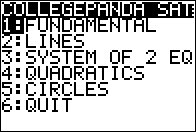The program consists of 5 main components:

• Fundamentals: basic math operations you may encounter on the exam
• Lines: slope, $$x$$-intercept, $$y$$-intercept, standard form
• Systems of Two Equations: solves systems of 2 equations in standard form
• Quadratics: quadratic formula (roots), vertex form, vertex, $$y$$-intercept, discriminant
• Circles: arc length, area of a sector, equation of a circle, center, radius, whether a point is inside a circle

#### Fundamentals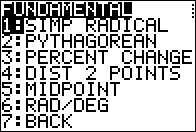Features:

• Pythagorean theorem
• Percent change
• Distance between 2 points
• Midpoint

#### Lines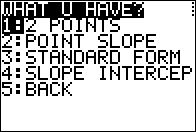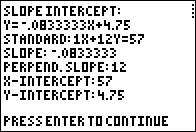Input any of the following: 2 points A point and a slope Standard form of a line Slope-intercept form of a line to get all of the following: The slope-intercept form of the line The standard form of the line The slope of the line The slope of a perpendicular line The $$x$$-intercept of the line The $$y$$-intercept of the line

#### Systems of Two Equations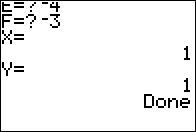• Solves any system of two equations expressed in $$ax + by = c$$ and $$dx + ey = f$$ form
• Indicates whether the system has infinite solutions
• Indicates whether the system has no solutions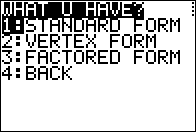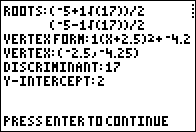Input any of the following: Standard form of a quadratic: $$ax^2 + bx + c$$ Vertex form of a quadratic: $$a(x + b)^2 + c$$ Factored form of a quadratic: $$a(x + b)(x + c)$$ to get all of the following: The roots/solutions (does the quadratic formula for you) Vertex form (completes the square for you) The vertex The discriminant The $$y$$-intercept

#### Circles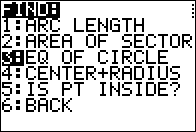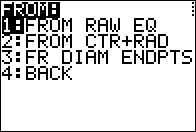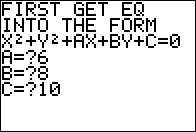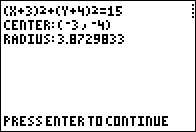• Arc length
• Area of a sector
• Equation of a circle from a raw equation ($$x^2 + y^2 + ax + by + c = 0$$)
• Equation of a circle from its center and radius
• Equation of a circle from the endpoints of a diameter
• The center and radius of a circle from its equation
• Checks whether a point is inside or outside a circle in the coordinate plane

### Companion Calculator Workbook

It's not enough to just have a program on your calculator. You need to know how and when to use it. This workbook, which contains exercises based on past SAT questions, will teach you how to use the program and for which question types.Chapters:

1. The Basics (based on part 1 of the video series)
2. Evaluating Expressions (based on part 2 of the video series)
3. Graphing (based on part 3 of the video series)
4. Systems of Equations (based on part 4 of the video series)
5. Lines (based on part 5 of the video series)
6. Fundamental Programs (based on part 6 of the video series)
7. Quadratics (based on part 6 of the video series)
8. Circles (based on part 6 of the video series)